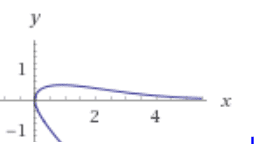# Find the area enclosed by the curve y = x csch(x+y)

• I
• Saracen Rue

#### Saracen Rue

TL;DR Summary
Determine the area enclosed by the curve ##y=x \text{ csch}(x+y)## and the positive ##x##-axis
The title and summary pretty much say it all. I was wondering if it's possible to accurately determine the area enclosed by the curve ## y=x \text{ csch}(x+y)## and the ##x##-axis?

I first tried solving for ##y## and then ##x##, however it doesn't appear possible to solve for either variable. I then recognised that the equation can be represented as a nested function, such that: $$f(x)=x \text{ csch}(x+x\text{ csch}(x+x\text{ csch}(x+x\text{ csch}(x+...))))$$

Through this I was able to use Desmos Graphing calculator to help me estimate the enclosed area to by integrating ##f(x)## from ##0## to ##\infty##. However, the imbeded nature of the function made it hard to properly find a definitive solution and I could only gather that the area appears to be approaching ##1.5## square units, although this might be inaccurate and it could be simply approaching a number near ##1.5##.

How would I go about accurately determining this area?
Any help is greatly appreciated.

Last edited by a moderator:

Summary:: Determine the area enclosed by the curve ##y=x \text{ csch}(x+y)## and the positive ##x##-axis

The title and summary pretty much say it all. I was wondering if it's possible to accurately determine the area enclosed by the curve ## y=x \text{ csch}(x+y)## and the ##x##-axis?

I first tried solving for ##y## and then ##x##, however it doesn't appear possible to solve for either variable. I then recognised that the equation can be represented as a nested function, such that: $$f(x)=x \text{ csch}(x+x\text{ csch}(x+x\text{ csch}(x+x\text{ csch}(x+...))))$$

Through this I was able to use Desmos Graphing calculator to help me estimate the enclosed area to by integrating ##f(x)## from ##0## to ##\infty##. However, the imbeded nature of the function made it hard to properly find a definitive solution and I could only gather that the area appears to be approaching ##1.5## square units, although this might be inaccurate and it could be simply approaching a number near ##1.5##.

How would I go about accurately determining this area?
Any help is greatly appreciated.
Your equation is equivalent to ##F(x, y) = 0##, with ##F(x, y) = x - xcsch(x + y)##. As such, the graph of F is a surface in ##\mathbb R^3##, and the equation you're working with is the cross-section of the surface in the x-y plane, i.e., where z = 0.
As far as how you would graph F(x, y) = 0, nothing comes to mind, except possibly calculating function values at a bunch of points. There are going to be discontinuities at points where ##x + y = k\pi##, k an integer.

As far as how you would graph F(x, y) = 0, nothing comes to mind
I think that was already done. Wolfram plots:Re: points where x+y=kπ ? it's hyperbolic: $$y = {x\over \sinh(x+y)}$$

I think that was already done. Wolfram plots:
View attachment 261445

Re: points where x+y=kπ ? it's hyperbolic: $$y = {x\over \sinh(x+y)}$$
Sorry for not being clear in the OP. You're right, I do already have the graph of ##y=x \text{ csch}(x+y)## and I'm just trying to find the area enclosed by the cruve and the ##x##-axis

Edit: Do you think it would be possible to use WolframAlpha's nest function to create and integrate a heavily nested form of ##y=x\text{ csch}(x+x\text{ csch}(x+...))## to allow for a better estimation of the area? I tried doing it myself but I'm not the most familiar with the language and could only seem to get it to nest every variable ##x## with ##y(x)##

Last edited: